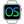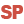Home |Sign in | EnglishHelpRectanglePresentationExampleWLanguage properties that can be used with the Rectangle typeAssigning a Rectangle variableAssigning an array of RectanglesComparison and serializationNotesSee alsoCharacter stringsConverting C typesCurrenciesDateDateTimeDurationJSONNumericOperations available for the dates, times and durations, ...RealsRectangleThe "Buffer" typeThe Variant typeThe different types of dataThe different types of integersTimeUUID
• WLanguage properties that can be used with the Rectangle type
• Assigning a Rectangle variable
• Assigning an array of Rectangles
• Comparison and serialization
• Notes
WINDEVWEBDEVWINDEV MobileOthersRectangle (Type of variable)
In french: Rectangle
A Rectangle variable is a structured type that is used to represent a rectangle (X, Y, Width, Height) in 2 dimensions.
Example
MyRectangle is Rectangle
MyRectangle..X = 280
MyRectangle..Y = 54
MyRectangle..Width = 50
MyRectangle..Height = 150
Syntax

Declaring and initializing a rectangle

<Rectangle Name> is Rectangle
<Rectangle Name>:
Name of the Rectangle variable to declare.
Remarks

WLanguage properties that can be used with the Rectangle type

The initial value of a rectangle is set to (0,0,0,0).
The following properties can be used to handle a Rectangle variable:
Property name Effect Height Height of the rectangle. This coordinate corresponds to a signed 4-byte integer. Width Width of the rectangle. This coordinate corresponds to a signed 4-byte integer. X X coordinate of the top left point. This coordinate corresponds to a signed 4-byte integer. Y Y coordinate of the top left point. This coordinate corresponds to a signed 4-byte integer.

Assigning a Rectangle variable

A Rectangle variable can be assigned with:
• another Rectangle variable.
For example:
MyRectangle is Rectangle
MyRectangle..X = 280
MyRectangle..Y = 54
MyRectangle..Width = 50
MyRectangle..Height = 150
MyRectangle1 is Rectangle = MyRectangle
• an array of four values that can be converted into integer.
For example:
MyRectangle is Rectangle
MyRectangle = [280, 54, 50, 150]
• two Point variables (corresponding respectively to the top left corner and to the bottom right corner of the rectangle).
For example:
MyRectangle is Rectangle
MyRectangle = [[280, 54], [330, 204]]

Assigning an array of Rectangles

An array of rectangles can be assigned with:
• an array of values containing rectangles.
For example:
ArrayOfRectangles is array of Rectangles
ArrayOfRectangles = [MyRectangle1, MyRectangle2]
• an array of four values that can be converted into integer.
For example:
ArrayOfRectangles is array of Rectangles
ArrayOfRectangles = [[1,2,3,4], [5,6,7,8]

Comparison and serialization

• Comparison: The comparison operators = and <> are available.
• Serialization: The binary, XML and JSON serializations are available.
Caution: During an XML serialization, the xmlAttribute constant cannot be used on a Rectangle class member or on a Rectangle structure member.

Notes

• A Rectangle variable can be stored in a variant.
• A Rectangle variable is never null:
• The "RectangleVariable = NULL" condition always returns False.
• The "RectangleVariable <> NULL" condition always returns True.
• You cannot reference a Rectangle variable.
The "RectangleVariable2 <- RectangleVariable1" syntax triggers an error.
• You cannot force the copy of a Rectangle variable.
The "RectangleVariable2 <= RectangleVariable1" syntax triggers an error.
• You have the ability to add a Point variable and a Rectangle variable. This operation is used to perform a relocation of the rectangle.
• You have the ability to subtract a Point variable to a Rectangle variable. This operation is used to perform a relocation of the rectangle.
• The Rectangle variables can be passed to the system APIs.
Minimum version required
• Version 21